Support the Monkey! Tell All your Friends and TeachersHome MonkeyNotes Printable Notes Digital Library Study Guides Study Smart Parents Tips College Planning Test Prep Fun Zone Help / FAQ How to Cite Request a New Title

Example A random sample of 400 workers from the Lorson and Lorson was drawn for estimating the average salary. The sample mean was \$ 800 and S.D. was \$100. Find 95% confidence interval.

Solution: 95% confidence limits are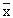± 1.96 S.E.

Now S.E. =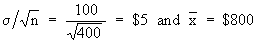Therefore we have± 1.96 S. E. = 800 ± 1.96 (5)

i.e. 800 - 1.96(5) = \$ 809 + 0

800 + 1.96(5) = \$ 890

Population proportion: For population proportion the limits are

1)  P' ± 1.96 S.E. at 95% confidence level.

2)  P' ± 2.58 S.E. at 99% confidence level.

3)  P' ± 3 S.E. at 99.73% confidence level.

When, instead of proportion, if we required the number in the size N of the population, from the sample of size 'n' the intervals are just multiplied by N.

i.e. N ( P' ± 1.96 S.E. ) for 9.5% confidence level etc.Your browser does not support the IFRAME tag.

Example A dice is thrown 9000 times and a throw of 5 or 6 considered success, was obtained 3240 times. Show that the dice is not unbiased and find the limits between which the probability of a throw of 5 or 6 lies.

Solution: The null hypothesis is that the dice is not unbiased.

In notation : Ho : Po = 0.5 i.e. Ha : Po ¹ 0.5

Po = 0.5 then qo = 0.5, n = 9000

Also, sample proportion =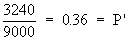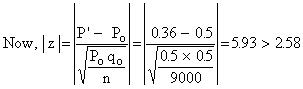Thus Ho is rejected i.e. the dice is not unbiased. The 99.73% confidence limits are P' ± 3 S.E. where P' = 0.36 and

S.E.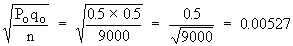Therefore, P' ± 3 S.E. = 0.36 ± 0.00527.

i.e. 0.36 - 0.00527 = 0.35473 to

0.36 + 0.00527 = 0.36527

Index

8.1 Population
8.2 Sample
8.3 Parameters and Statistic
8.4 Sampling Distribution
8.5 Sampling Error
8.6 Central Limit Theorem
8.7 Critical Region
8.8 Testing of Hypothesis
8.9 Errors in Tesitng of Hypothesis
8.10 Power o a Hypothesis Test
8.11 Sampling of Variables
8.12 Sampling of Attributes
8.13 Estimation
8.14 Testing the Difference Between Means
8.15 Test for Difference Between Proportions
8.16 Two Tailed and one Tailed Tests
8.17 Test of Significance for Small Samples
8.18 Students t-distribution
8.19 Distribution of 't' for Comparison of Two Samples Means Independent Samples
8.20 Testing Difference Between Mens of Two Samples Dependent Samples or Matched Paired Observations
8.21 Chi-Square
8.22 Sampling Theory of Correlation
8.23 Sampling Theory of Regression

Chapter 1Search: All Products Books Popular Music Classical Music Video DVD Toys & Games Electronics Software Tools & Hardware Outdoor Living Kitchen & Housewares Camera & Photo Cell Phones Keywords: